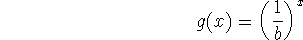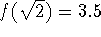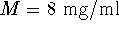Next: About this document Up: Exponentials - Part 1 Previous: Exponential decay and effective

## Exercises

1. Suppose that b satisfies b > 1. Explain the relationship between the graphs ofand2. Suppose that f(x) is an exponential function that satisfies. Find the value of b so thatand compute the value of f(2).

3. Suppose that the population of a certain bacteria can be modeled by an exponential function. In a particular experiment, the number of bacteria was 10,000 at t=0. Four hours later, the number of bacteria was 250,000. Suppose a second experiment is performed under the same conditions, but the number of bacteria at t=0 is only 2000. Show, using the equation for exponential growth, that the predicted number of bacteria after four hours in this second experiment is 50,000.
4. Suppose that for a certain drug, the following results were obtained. Immediately after the drug was administered, the concentration was 3.3 mg/ml. Six hours later, the concentration had dropped to 1.55 mg/ml. Determine the value of k for this drug.
5. Suppose that for the drug in the previous exercise, the maximum safe level isand the minimum effective level is. What is the maximum possible time between doses for this drug?

William W. Farr
Fri Jan 31 14:58:36 EST 1997